CBSE Class 12 Sample Paper for 2019 Boards

Class 12
Solutions of Sample Papers and Past Year Papers - for Class 12 Boards

Question 16 (OR 1 st question)

If y = x sin ⁡x + sin⁡ (x x ), find dy/dx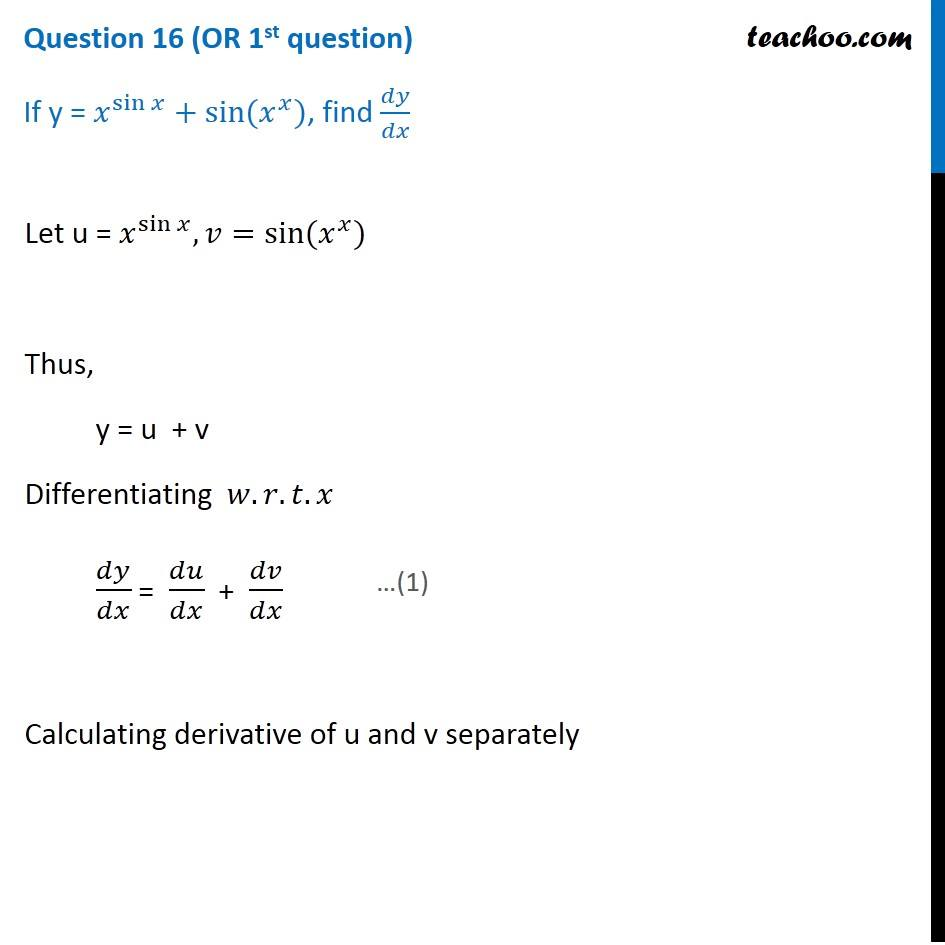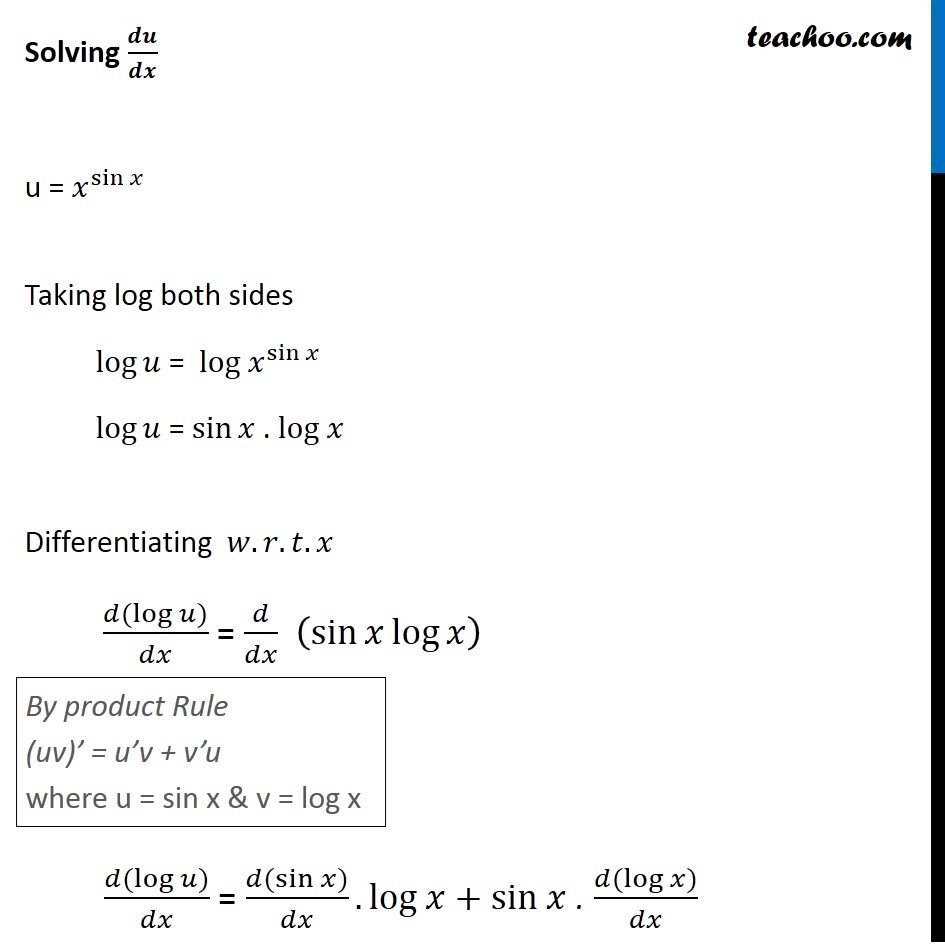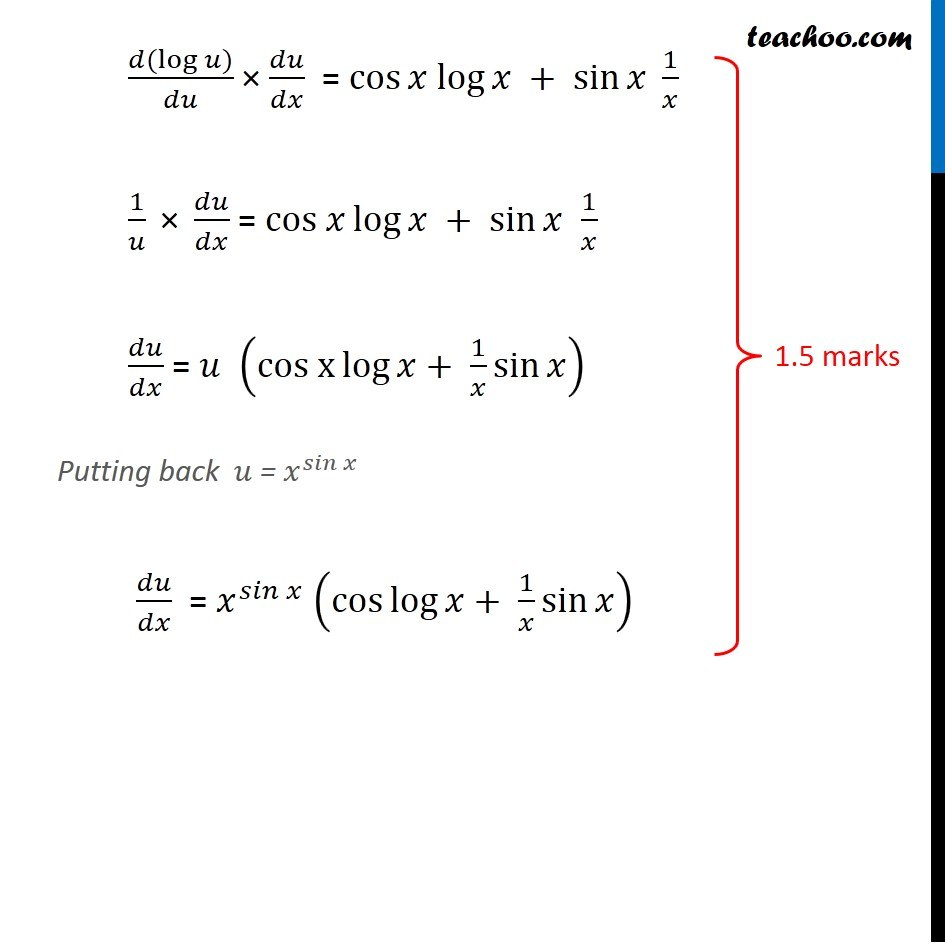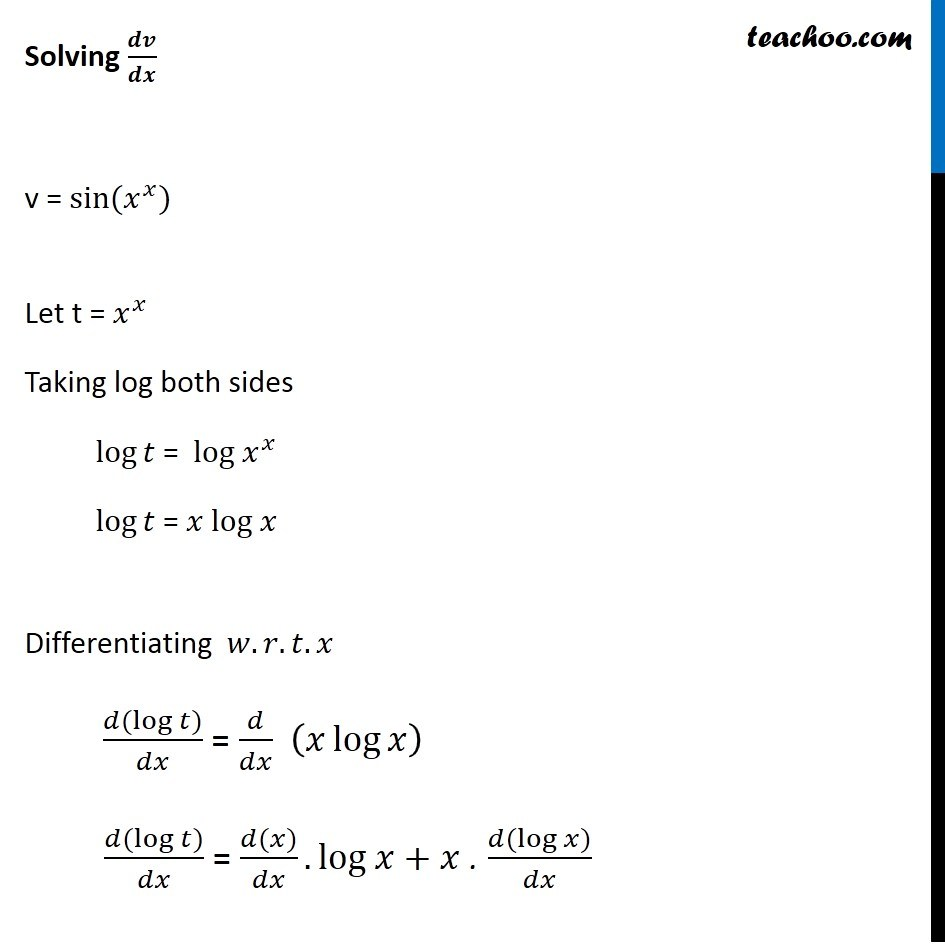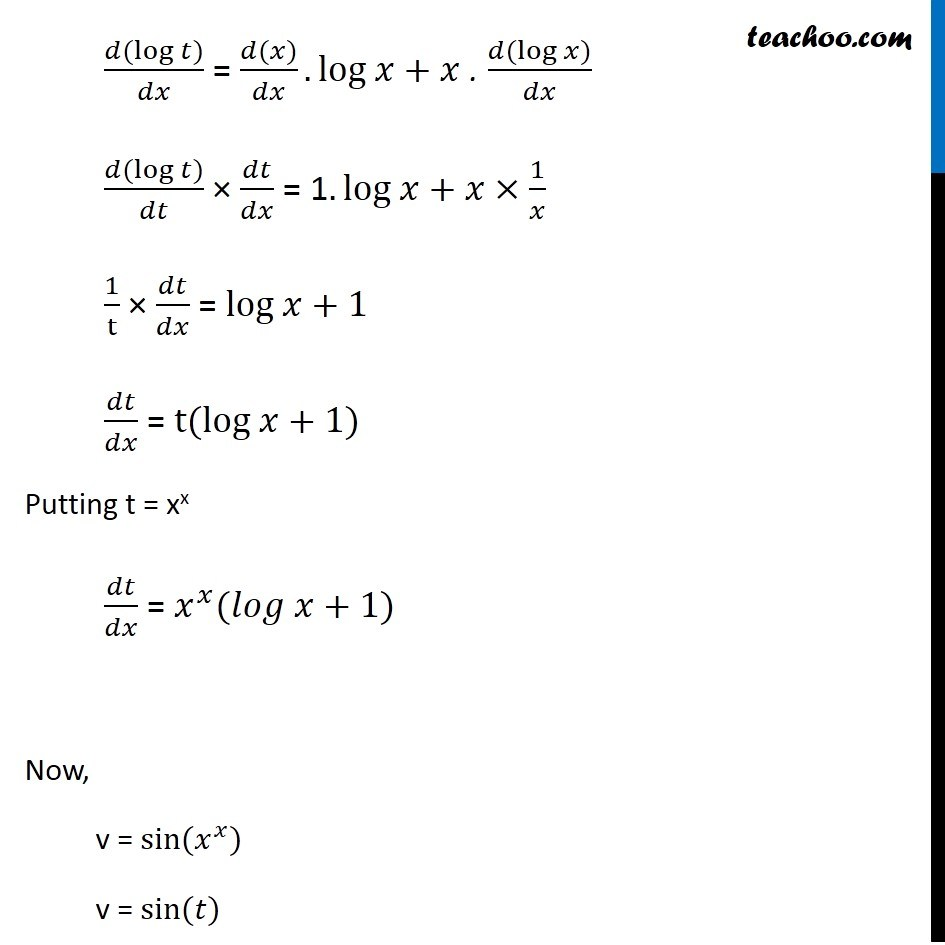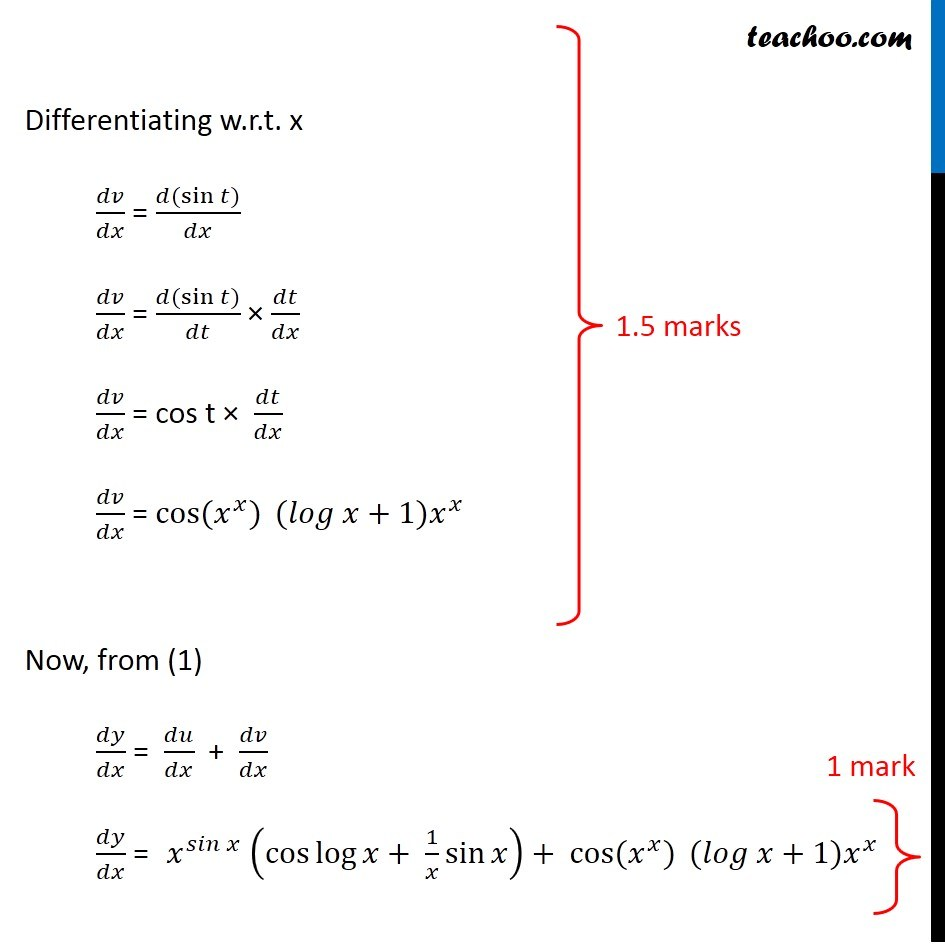Learn in your speed, with individual attention - Teachoo Maths 1-on-1 Class

### Transcript

Question 16 (OR 1st question) If y = 𝑥^sin⁡𝑥 +sin⁡〖(𝑥^𝑥)〗, find 𝑑𝑦/𝑑𝑥 Let u = 𝑥^sin⁡𝑥 , 𝑣=sin⁡〖(𝑥^𝑥)〗 Thus, y = u + v Differentiating 𝑤.𝑟.𝑡.𝑥 𝑑𝑦/𝑑𝑥 = 𝑑𝑢/𝑑𝑥 + 𝑑𝑣/𝑑𝑥 Calculating derivative of u and v separately Solving 𝒅𝒖/𝒅𝒙 u = 𝑥^sin⁡𝑥 Taking log both sides log⁡𝑢 = log 𝑥^sin⁡𝑥 log⁡𝑢 = sin⁡𝑥 . log 𝑥 Differentiating 𝑤.𝑟.𝑡.𝑥 (𝑑(log⁡〖𝑢)〗)/𝑑𝑥 = 𝑑/𝑑𝑥 (sin⁡〖𝑥 log⁡𝑥 〗 ) By product Rule (uv)’ = u’v + v’u where u = sin x & v = log x (𝑑(log⁡〖𝑢)〗)/𝑑𝑥 = (𝑑(sin⁡𝑥))/𝑑𝑥.log 𝑥+sin 𝑥 . (𝑑(log⁡𝑥))/𝑑𝑥 (𝑑(log⁡〖𝑢)〗)/𝑑𝑢 × 𝑑𝑢/𝑑𝑥 = cos⁡𝑥 log⁡𝑥 + sin⁡𝑥 1/𝑥 1/𝑢 " × " 𝑑𝑢/𝑑𝑥 = cos 𝑥⁡log⁡𝑥 + sin⁡𝑥 1/𝑥 𝑑𝑢/𝑑𝑥 = 𝑢 (〖cos x〗⁡〖log⁡〖𝑥+ 1/𝑥〗 sin⁡𝑥 〗 ) Putting back 𝑢 = 𝑥^𝑠𝑖𝑛⁡𝑥 𝑑𝑢/𝑑𝑥 = 𝑥^𝑠𝑖𝑛⁡𝑥 (cos⁡〖log⁡〖𝑥+ 1/𝑥〗 sin⁡𝑥 〗 ) Solving 𝒅𝒗/𝒅𝒙 v = sin⁡〖(𝑥^𝑥)〗 Let t = 𝑥^𝑥 Taking log both sides log⁡𝑡 = log 𝑥^𝑥 log⁡𝑡 = 𝑥 log 𝑥 Differentiating 𝑤.𝑟.𝑡.𝑥 (𝑑(log⁡〖𝑡)〗)/𝑑𝑥 = 𝑑/𝑑𝑥 (𝑥 log⁡𝑥 ) (𝑑(log⁡〖𝑡)〗)/𝑑𝑥 = (𝑑(𝑥))/𝑑𝑥.log 𝑥+𝑥 . (𝑑(log⁡𝑥))/𝑑𝑥 (𝑑(log⁡〖𝑡)〗)/𝑑𝑥 = (𝑑(𝑥))/𝑑𝑥.log 𝑥+𝑥 . (𝑑(log⁡𝑥))/𝑑𝑥 (𝑑(log⁡〖𝑡)〗)/𝑑𝑡 × 𝑑𝑡/𝑑𝑥 = 1.log 𝑥+𝑥×1/𝑥 1/t × 𝑑𝑡/𝑑𝑥 = log 𝑥+1 𝑑𝑡/𝑑𝑥 = t(log 𝑥+1) Putting t = xx 𝑑𝑡/𝑑𝑥 = 𝑥^𝑥 (𝑙𝑜𝑔 𝑥+1) Now, v = sin⁡〖(𝑥^𝑥)〗 v = sin⁡〖(𝑡)〗 Differentiating w.r.t. x 𝑑𝑣/𝑑𝑥 = (𝑑(sin⁡𝑡))/𝑑𝑥 𝑑𝑣/𝑑𝑥 = (𝑑(sin⁡𝑡))/𝑑𝑡 × 𝑑𝑡/𝑑𝑥 𝑑𝑣/𝑑𝑥 = cos t × 𝑑𝑡/𝑑𝑥 𝑑𝑣/𝑑𝑥 = cos⁡〖(𝑥^𝑥)〗 (𝑙𝑜𝑔 𝑥+1)𝑥^𝑥 Now, from (1) 𝑑𝑦/𝑑𝑥 = 𝑑𝑢/𝑑𝑥 + 𝑑𝑣/𝑑𝑥 𝑑𝑦/𝑑𝑥 = 𝑥^𝑠𝑖𝑛⁡𝑥 (cos⁡〖log⁡〖𝑥+ 1/𝑥〗 sin⁡𝑥 〗 )+ cos⁡〖〖(𝑥〗^𝑥)〗 (𝑙𝑜𝑔 𝑥+1)𝑥^𝑥